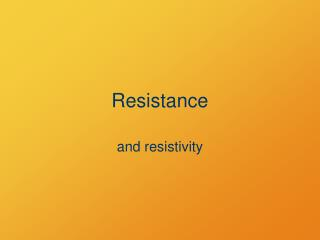DownloadDownload PresentationResistance

# Resistance

Télécharger la présentation## Resistance

- - - - - - - - - - - - - - - - - - - - - - - - - - - E N D - - - - - - - - - - - - - - - - - - - - - - - - - - -
##### Presentation Transcript

1. Resistance and resistivity

2. Current • Current is sort of a vector • Direction is constrained by conductor • Restricted to forward or backward (+ or –)

3. Resistance • Current does not flow unhindered • Electrical resistance is analogous to friction or drag • Expressed as potential needed to maintain a current

4. Ohm’s Law DV I = R I = current DV = voltage = electric potential drop R = resistance Unit of resistance : V / A = ohm (W)

5. Voltage Causes Current Potential drop is the cause. Current is the effect. Resistance reduces the effect of potential.

6. Does it Work? • Approximation of varying utility:R is independent of DV and I • When true, the material is ohmic

7. Poll Question If you want to increase the current through a resistor, you need to • Increase the resistance or voltage. • Decrease the resistance or voltage. • Increase the resistance or decrease the voltage. • Decrease the resistance or increase the voltage.

8. Ohm’s Law Rearranged If you know two, you can find the third. DV DV I = R = DV= IR R I I = current DV = potential R = resistance

9. Example A 1.5-V battery powers a light bulb with a resistance of 9 W. What is the current through the bulb? Ohm’s Law I = V / R V = 1.5 V; R = 9 W I = (1.5 V ) / (9 V/A) = 1/6 A

10. Resistivity For current through a cylinder: L A • Longer L greaterR. • Greater A smallerR. • More resistive material  bigger R.

11. Resistivity • R = rL/A • ris Resistivity • Unit: ohm·meter = Wm • More or less constant depending on material, conditions

12. Resistivity • Intensive quantity • Does not depend on the amount of material, only its conditions • Predictive value when mostly constant (ohmic)

13. Resistivities vary widely

14. Example The resistivity of copper is 1.710–8Wm. What is the resistance of a 100-km length of copper wire that is 1/4” in diameter?

15. Classes of Conductors • How resistivity changes with temperature a = temperature coefficient of resistivity

16. Classes of Conductors • How resistivity changes with temperature

17. Power dissipated by a resistor

18. Electric Power Potential is energy per charge:V = DE/ q Current is charge per time:I= q /Dt So, (potential times current) = (energy per time) = power Power = VI

19. Group Work Power P = VI and V = IR. Using these, show that: • P = I2R • P = V2/R